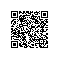C语言面试题分析之五

# 一.    关于C语言指针的经典面试题

a) 一个整型数（An integer
b)

c)

to an integer

d)

e)

integers

f)

g)

nction that takes an integer as an argument and returns an integer

h)

eturn an integer

a) int a; // An integer
b) int *a; // A pointer to an integer
c) int **a; // A pointer to a pointer to an integer
d) int a; // An array of 10 integers
e) int *a; // An array of 10 pointers to integers
f) int (*a); // A pointer to an array of 10 integers
g) int (*a)(int); // A pointer to a function a that takes an integer argument
and returns an integer
h) int (*a)(int); // An array of 10 pointers to functions that take an int
eger argument and return an integer使用钉钉扫一扫加入圈子
+ 订阅